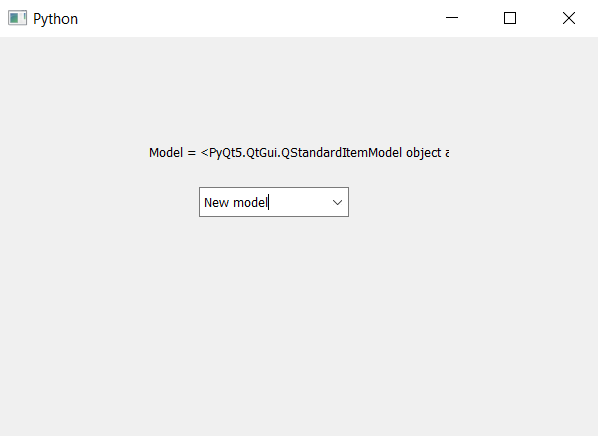Related Articles

# PyQt5 – How to get the model of ComboBox

• Last Updated : 22 Apr, 2020

In this article we will see how we can get model of the combo box. Model is used to set the items of the combo box. In order to set the model we will use `setModel` method. If we add model after the insertion of items it will clear all the elements so model should be added before any insertion of elements. In order to get the model we use `model` method.

Syntax : combo_box.model()

Argument : It takes no argument

Return : It returns QStandardItemModel object

Below is the implementation –

 `# importing libraries``from` `PyQt5.QtWidgets ``import` `*` `from` `PyQt5 ``import` `QtCore, QtGui``from` `PyQt5.QtGui ``import` `*` `from` `PyQt5.QtCore ``import` `*` `import` `sys`` ` ` ` `class` `Window(QMainWindow):`` ` `    ``def` `__init__(``self``):``        ``super``().__init__()`` ` `        ``# setting title``        ``self``.setWindowTitle(``"Python "``)`` ` `        ``# setting geometry``        ``self``.setGeometry(``100``, ``100``, ``600``, ``400``)`` ` `        ``# calling method``        ``self``.UiComponents()`` ` `        ``# showing all the widgets``        ``self``.show()`` ` `    ``# method for widgets``    ``def` `UiComponents(``self``):`` ` `        ``# creating a combo box widget``        ``self``.combo_box ``=` `QComboBox(``self``)`` ` `        ``# setting geometry of combo box``        ``self``.combo_box.setGeometry(``200``, ``150``, ``150``, ``30``)`` ` `        ``# geek list``        ``geek_list ``=` `[``"Sayian"``, ``"Super Saiyan"``, ``"Super Sayian 2"``,``                                               ``"Super Sayian B"``]`` ` `        ``# making it editable``        ``self``.combo_box.setEditable(``True``)`` ` `        ``# adding list of items to combo box``        ``self``.combo_box.addItems(geek_list)`` ` `        ``# creating a model``        ``model ``=` `QtGui.QStandardItemModel(``0``, ``1``)`` ` `        ``# creating a item``        ``item ``=` `QtGui.QStandardItem(``"New model"``)`` ` `        ``# adding item to the model``        ``model.appendRow(item)`` ` `        ``# setting model to the combo box``        ``self``.combo_box.setModel(model)`` ` `        ``# getting the model``        ``get_model ``=` `self``.combo_box.model()`` ` `        ``# creating label to show model``        ``label ``=` `QLabel(``"Model = "` `+` `str``(get_model), ``self``)`` ` `        ``# setting geometry to the label``        ``label.setGeometry(``150``, ``100``, ``300``, ``30``)`` ` ` ` `# create pyqt5 app``App ``=` `QApplication(sys.argv)`` ` `# create the instance of our Window``window ``=` `Window()`` ` `# start the app``sys.exit(App.``exec``())`

Output :Attention geek! Strengthen your foundations with the Python Programming Foundation Course and learn the basics.

To begin with, your interview preparations Enhance your Data Structures concepts with the Python DS Course. And to begin with your Machine Learning Journey, join the Machine Learning – Basic Level Course

My Personal Notes arrow_drop_up